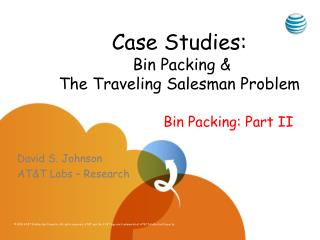# Case Studies: Bin Packing & The Traveling Salesman Problem - PowerPoint PPT PresentationDownload PresentationCase Studies: Bin Packing & The Traveling Salesman Problem

Case Studies: Bin Packing & The Traveling Salesman ProblemDownload Presentation## Case Studies: Bin Packing & The Traveling Salesman Problem

- - - - - - - - - - - - - - - - - - - - - - - - - - - E N D - - - - - - - - - - - - - - - - - - - - - - - - - - -
##### Presentation Transcript

1. Case Studies: Bin Packing &The Traveling Salesman Problem Bin Packing: Part II David S. Johnson AT&T Labs – Research

2. Asymptotic Worst-Case Ratios • Theorem: R∞(FF) = R∞(BF) = 17/10. • Theorem: R∞(FFD) = R∞(BFD) = 11/9.

3. Average-Case Performance

4. Progress?

5. Progress:Faster Computers  Bigger Instances

6. Definitions

7. Definitions, Continued

8. Theorems for U[0,1]

9. Distributions U[0,u] Item sizes uniformly distributed in the interval (0,u], 0 < u < 1

10. Average Waste for BF under U(0,u]

11. Measured Average Waste for BF under U(0,.01]

12. Conjecture

13. FFD on U(0,u] u = .6 FFD(L) – s(L) u = .5 u = .4 N = Experimental Results from [Bentley, Johnson, Leighton, McGeoch, 1983]

14. FFD on U(0,u], u  0.5

15. FFD on U(0,u], u  0.5

16. FFD on U(0,u], 0.5  u  1 1984 – 2011?)

17. Discrete Distributions

18. Courcoubetis-Weber

19. y z (0,2,1) (1,0,2) (2,1,1) x (0,0,0)

20. Courcoubetis-Weber Theorem

21. A Flow-Based Linear Program

22. Theorem [Csirik et al. 2000] Note: The LP’s for (1) and (3) are both of size polynomial in B, not log(B), and hence “pseudo-polynomial”

23. U{6,8} U{12,16} U{3,4} U(0,¾] 1 2/3 1/3 0.00 0.25 0.50 0.75 1.00 Discrete Uniform Distributions

24. Theorem [Coffman et al. 1997] (Results analogous to those for the corresponding U(0,u])

25. Experimental Results for Best Fit0 ≤ u ≤ 1, 1 ≤ j ≤ k = 51 Averages of 25 trials for each distribution, N = 2,048,000

26. Average Waste under Best Fit(Experimental values for N = 100,000,000 and 200,000,000) Linear Waste [GJSW, 1993]

27. Average Waste under Best Fit(Experimental values for N = 100,000,000 and 200,000,000) [KRS, 1996] Holds for all j = k-2 [GJSW, 1993]

28. Average Waste under Best Fit(Experimental values for N = 100,000,000 and 200,000,000) Still Open [GJSW, 1993]

29. Theorem [Kenyon & Mitzenmacher, 2000]

30. Average wBF(L)/s(L) for U{j,85}

31. Average wBFD(L)/s(L) for U{j,85}

32. Averages on the Same Scale

33. The Discrete Distribution U{6,13}

34. ¾β 6 β/24 3 2 3 3 3 3 4 6 2 5 5 2 4 2 β/6 β/2 β/2 2 4 2 β/2 β/2 β/3 β/8 β/24 “Fluid Algorithm” Analysis: U{6,13} Size = 6 5 4 3 2 1 Amount = ββββββ Bin Type = Amount =

35. Expected Waste

36. Theorem[Coffman, Johnson, McGeoch, Shor, & Weber, 1994-2011]

37. Waste as a Function of j and k (mod 6)

38. K = 8641 = 26335 + 1

39. Beating BF and BFD in Theory

40. Plausible Alternative Approach

41. The Sum-of-Squares Algorithm (SS)

42. SS on U{j,100} for 1 ≤ j ≤ 99 BF for N = 10M SS for N = 100K SS(L)/s(L) SS for N = 1M SS for N = 10M j

43. Discrete Uniform Distributions II